# 9 3 Graphing Quadratic Functions Preview Warm Up

• Slides: 419 -3 Graphing Quadratic Functions Preview Warm Up California Standards Lesson Presentation9 -3 Graphing Quadratic Functions Warm Up Find the axis of symmetry. 1. y = 4 x 2 – 7 x = 0 2. y = x 2 – 3 x + 1 3. y = – 2 x 2 + 4 x + 3 x = 1 4. y = – 2 x 2 + 3 x – 1 Find the vertex. 5. y = x 2 + 4 x + 5 (– 2, 1) 7. y = 2 x 2 + 2 x – 8 6. y = 3 x 2 + 2 (0, 2)9 -3 Graphing Quadratic Functions California Standards 21. 0 Students graph quadratic functions and know that their roots are the x-intercepts. 23. 0 Students apply quadratic equations to physical problems, such as the motion of an object under the force of gravity.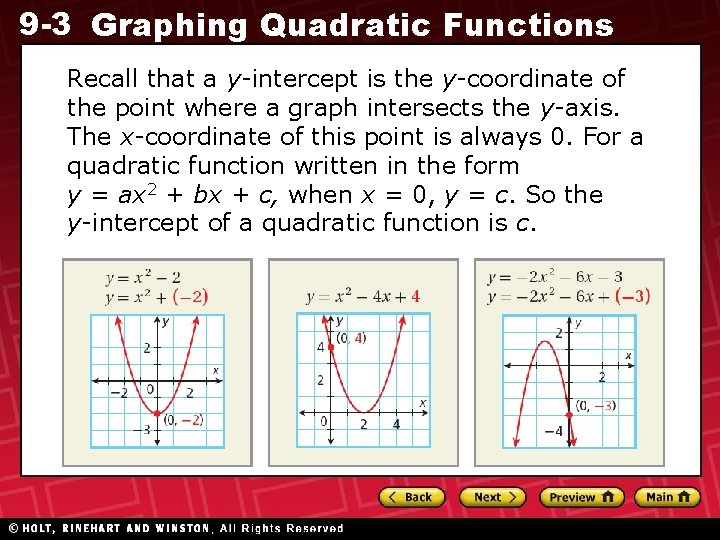9 -3 Graphing Quadratic Functions Recall that a y-intercept is the y-coordinate of the point where a graph intersects the y-axis. The x-coordinate of this point is always 0. For a quadratic function written in the form y = ax 2 + bx + c, when x = 0, y = c. So the y-intercept of a quadratic function is c.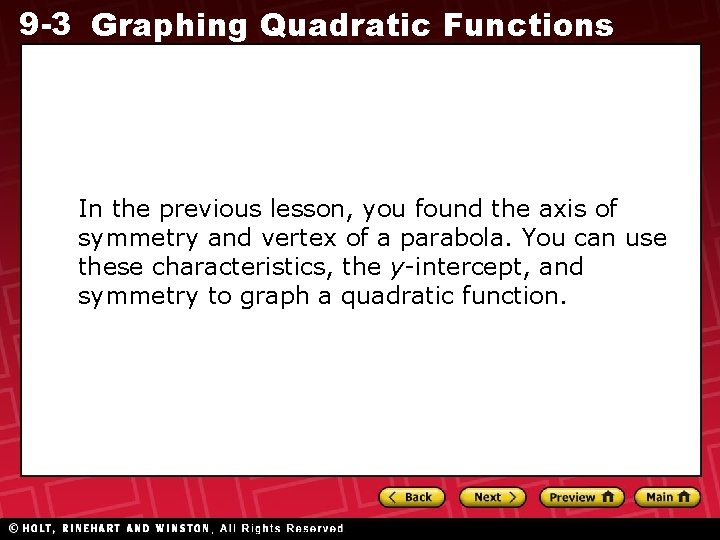9 -3 Graphing Quadratic Functions In the previous lesson, you found the axis of symmetry and vertex of a parabola. You can use these characteristics, the y-intercept, and symmetry to graph a quadratic function.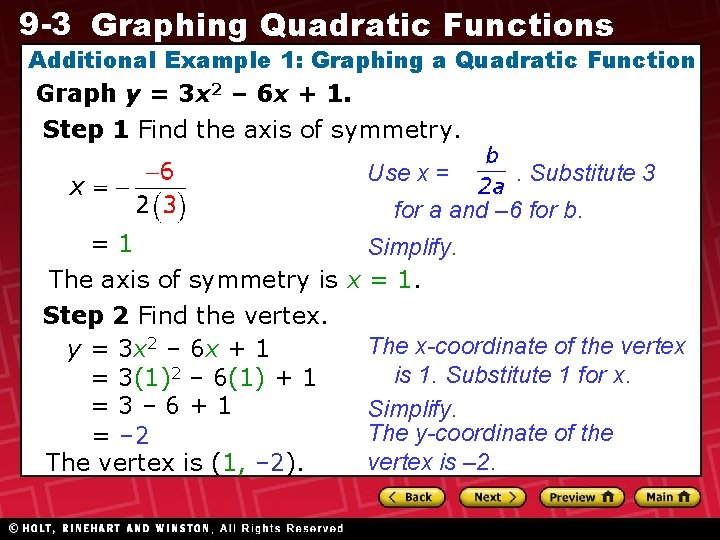9 -3 Graphing Quadratic Functions Additional Example 1: Graphing a Quadratic Function Graph y = 3 x 2 – 6 x + 1. Step 1 Find the axis of symmetry. Use x = . Substitute 3 for a and – 6 for b. =1 Simplify. The axis of symmetry is x = 1. Step 2 Find the vertex. The x-coordinate of the vertex y = 3 x 2 – 6 x + 1 is 1. Substitute 1 for x. = 3(1)2 – 6(1) + 1 =3– 6+1 Simplify. The y-coordinate of the = – 2 vertex is – 2. The vertex is (1, – 2).9 -3 Graphing Quadratic Functions Additional Example 1 Continued Step 3 Find the y-intercept. y = 3 x 2 – 6 x + 1 Identify c. The y-intercept is 1; the graph passes through (0, 1).9 -3 Graphing Quadratic Functions Additional Example 1 Continued Step 4 Find two more points on the same side of the axis of symmetry as the point containing the y-intercept. Since the axis of symmetry is x = 1, choose xvalues less than 1. Substitute Let x = – 2. x-coordinates. y = 3(– 1)2 – 6(– 1) + 1 y = 3(– 2)2 – 6(– 2) + 1 =3+6+1 = 12 + 1 Simplify. = 10 = 25 Let x = – 1. Two other points are (– 1, 10) and (– 2, 25).9 -3 Graphing Quadratic Functions Additional Example 1 Continued Graph y = 3 x 2 – 6 x + 1. Step 5 Graph the axis of Step 6 Reflect the points symmetry, the vertex, the point across the axis of symmetry. containing the y-intercept, and two Connect the points with a other points. smooth curve. (– 2, 25) x=1 (– 1, 10) (0, 1) (1, – 2)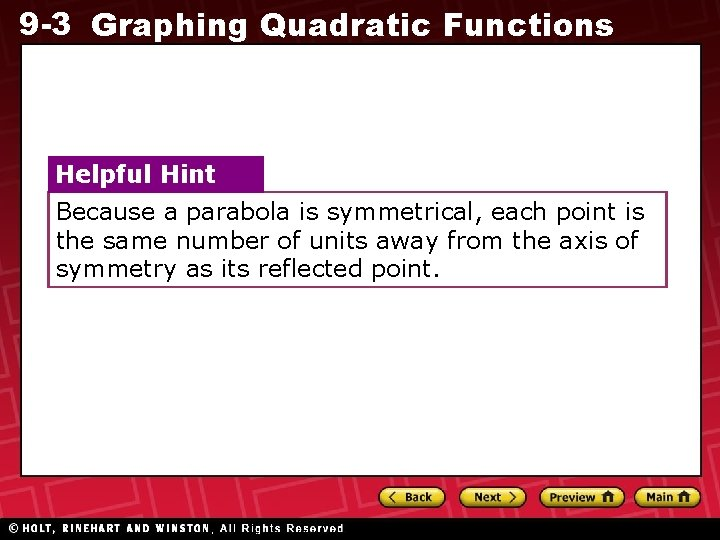9 -3 Graphing Quadratic Functions Helpful Hint Because a parabola is symmetrical, each point is the same number of units away from the axis of symmetry as its reflected point.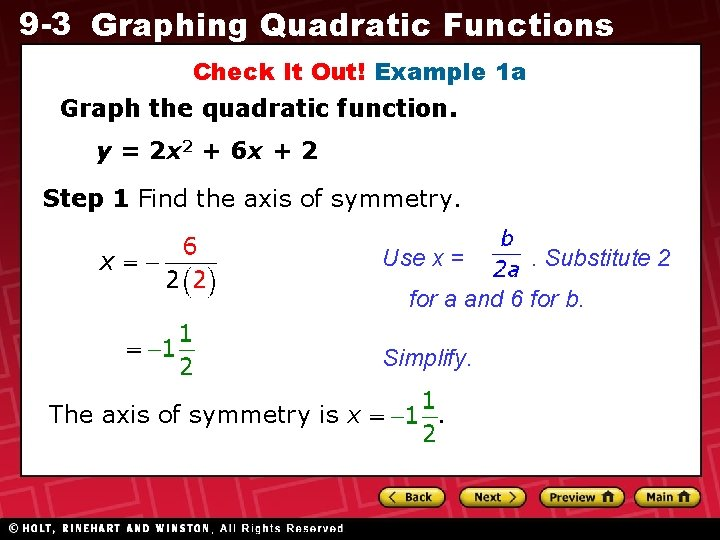9 -3 Graphing Quadratic Functions Check It Out! Example 1 a Graph the quadratic function. y = 2 x 2 + 6 x + 2 Step 1 Find the axis of symmetry. Use x = . Substitute 2 for a and 6 for b. Simplify. The axis of symmetry is x .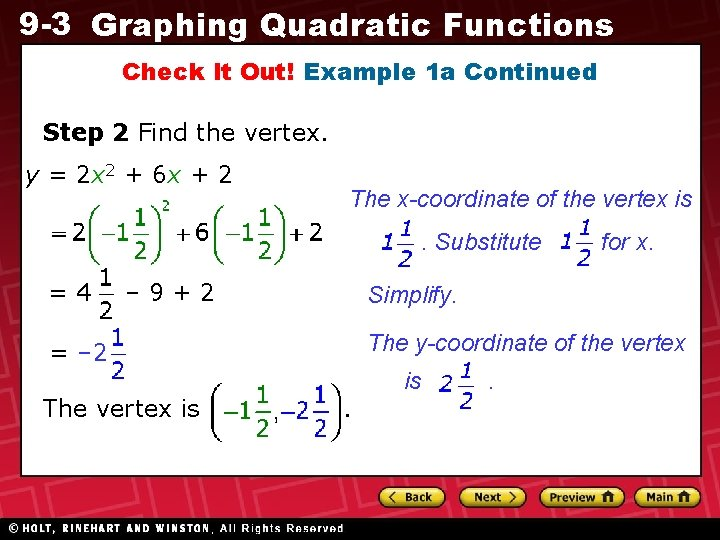9 -3 Graphing Quadratic Functions Check It Out! Example 1 a Continued Step 2 Find the vertex. y = 2 x 2 + 6 x + 2 The x-coordinate of the vertex is. Substitute =4 – 9+2 Simplify. The y-coordinate of the vertex = – 2 The vertex is for x. . is .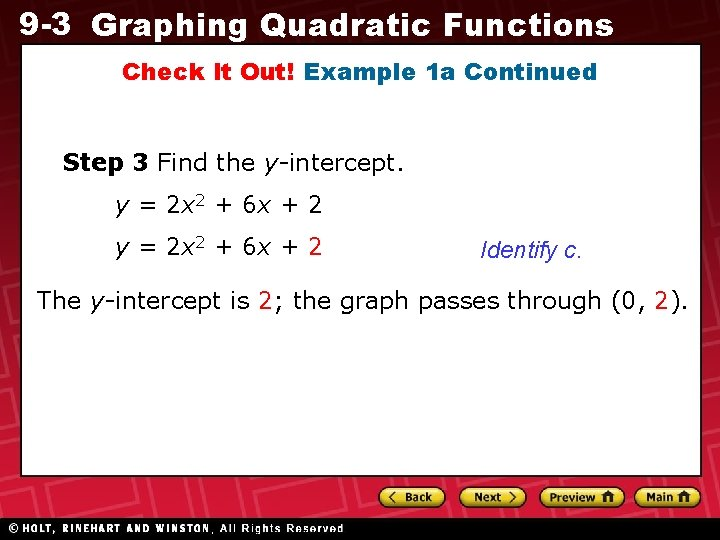9 -3 Graphing Quadratic Functions Check It Out! Example 1 a Continued Step 3 Find the y-intercept. y = 2 x 2 + 6 x + 2 Identify c. The y-intercept is 2; the graph passes through (0, 2).9 -3 Graphing Quadratic Functions Check It Out! Example 1 a Continued Step 4 Find two more points on the same side of the axis of symmetry as the point containing the y-intercept. Since the axis of symmetry is x = – 1 , choose x values greater than – 1. Let x = – 1 Let x = 1 y = 2(– 1)2 + 6(– 1) + 1 Substitute y = 2(1)2 + 6(1) + 2 x-coordinates. = 2 + 6 + 2 =2– 6+2 Simplify. = – 2 = 10 Two other points are (– 1, – 2) and (1, 10).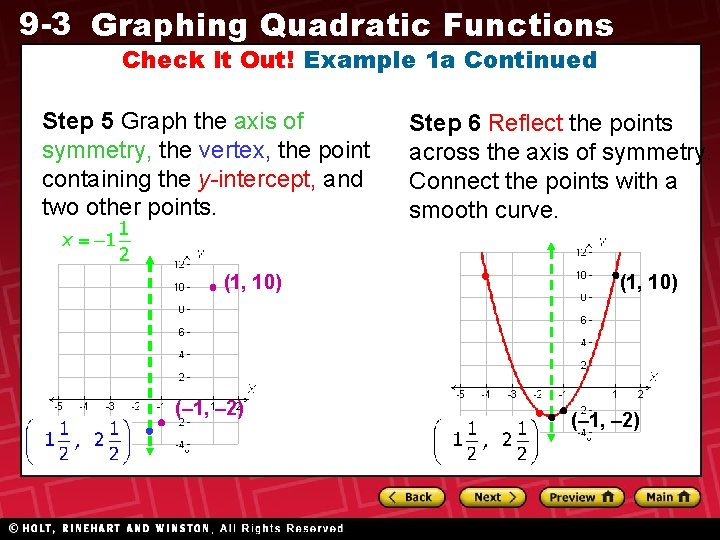9 -3 Graphing Quadratic Functions Check It Out! Example 1 a Continued Step 5 Graph the axis of symmetry, the vertex, the point containing the y-intercept, and two other points. (1, 10) (– 1, – 2) Step 6 Reflect the points across the axis of symmetry. Connect the points with a smooth curve. (1, 10) (– 1, – 2)9 -3 Graphing Quadratic Functions Check It Out! Example 1 b Graph the quadratic function. y + 6 x = x 2 + 9 y = x 2 – 6 x + 9 Rewrite in standard form. Step 1 Find the axis of symmetry. Use x = . Substitute 1 for a and – 6 for b. =3 Simplify. The axis of symmetry is x = 3.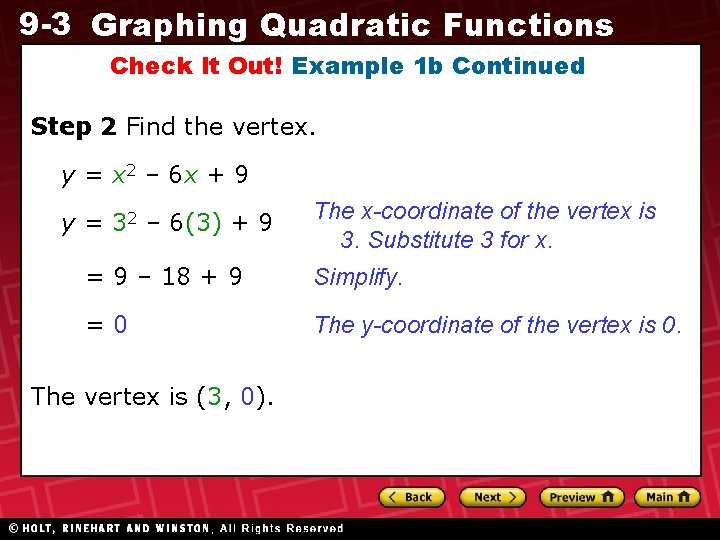9 -3 Graphing Quadratic Functions Check It Out! Example 1 b Continued Step 2 Find the vertex. y = x 2 – 6 x + 9 y = 32 – 6(3) + 9 The x-coordinate of the vertex is 3. Substitute 3 for x. = 9 – 18 + 9 Simplify. =0 The y-coordinate of the vertex is 0. The vertex is (3, 0).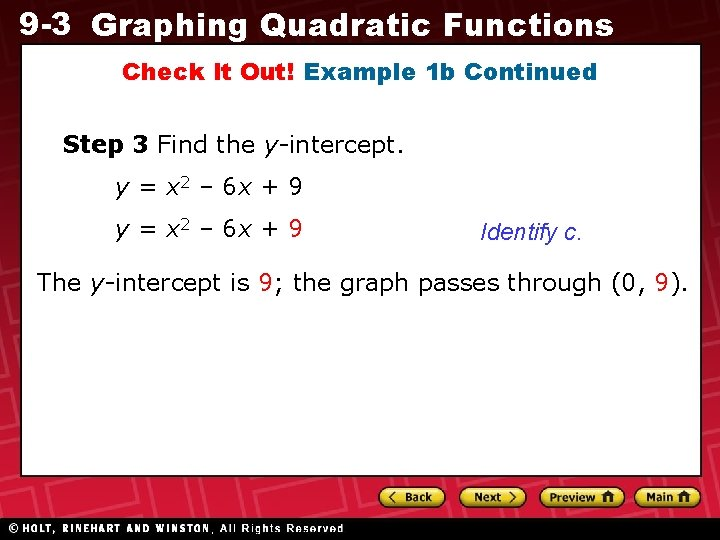9 -3 Graphing Quadratic Functions Check It Out! Example 1 b Continued Step 3 Find the y-intercept. y = x 2 – 6 x + 9 Identify c. The y-intercept is 9; the graph passes through (0, 9).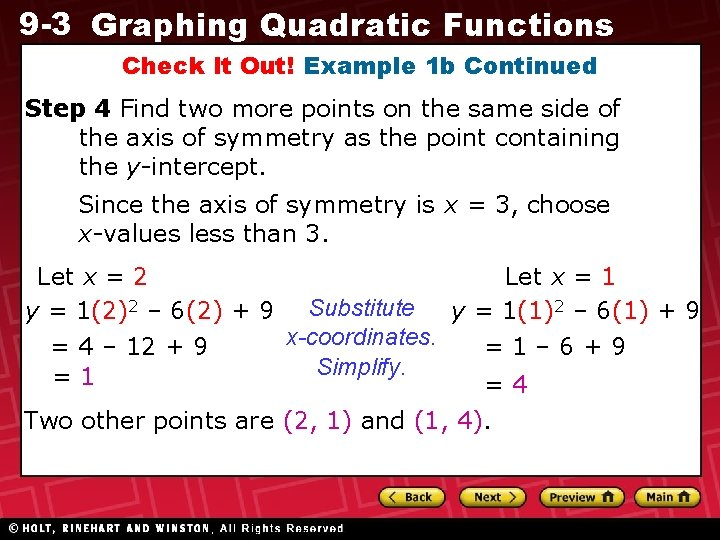9 -3 Graphing Quadratic Functions Check It Out! Example 1 b Continued Step 4 Find two more points on the same side of the axis of symmetry as the point containing the y-intercept. Since the axis of symmetry is x = 3, choose x-values less than 3. Let x = 2 Let x = 1 y = 1(2)2 – 6(2) + 9 Substitute y = 1(1)2 – 6(1) + 9 x-coordinates. = 4 – 12 + 9 =1– 6+9 Simplify. =1 =4 Two other points are (2, 1) and (1, 4).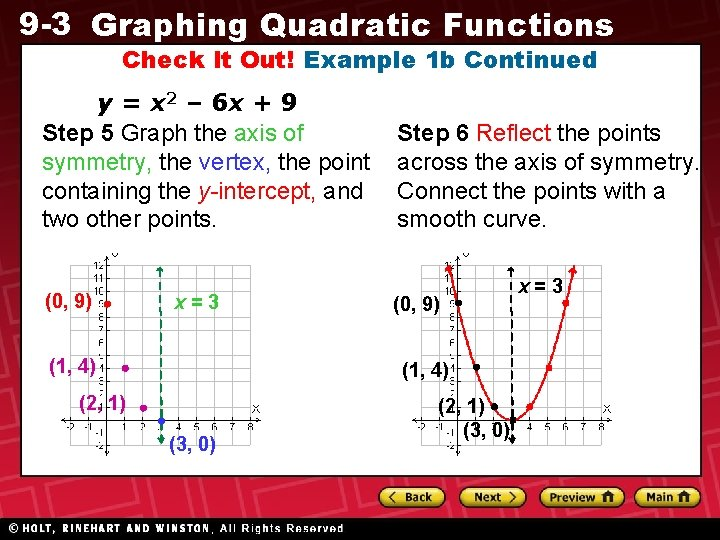9 -3 Graphing Quadratic Functions Check It Out! Example 1 b Continued y = x 2 – 6 x + 9 Step 5 Graph the axis of symmetry, the vertex, the point containing the y-intercept, and two other points. (0, 9) x=3 (1, 4) Step 6 Reflect the points across the axis of symmetry. Connect the points with a smooth curve. (0, 9) (1, 4) (2, 1) (3, 0) x=3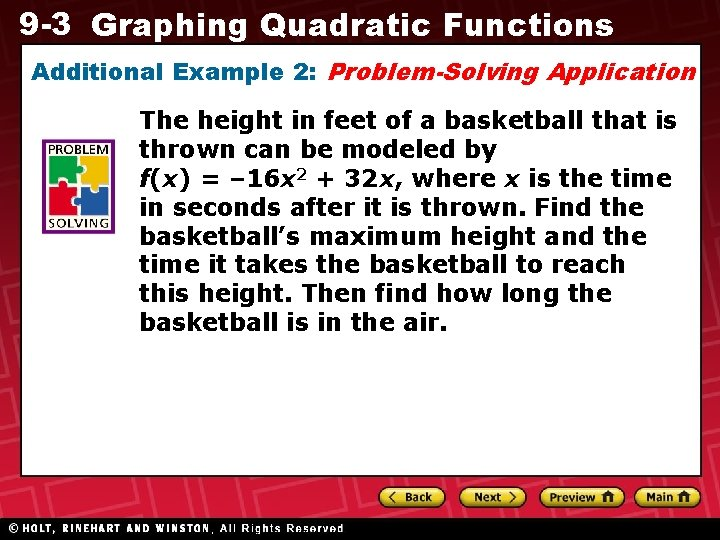9 -3 Graphing Quadratic Functions Additional Example 2: Problem-Solving Application The height in feet of a basketball that is thrown can be modeled by f(x) = – 16 x 2 + 32 x, where x is the time in seconds after it is thrown. Find the basketball’s maximum height and the time it takes the basketball to reach this height. Then find how long the basketball is in the air.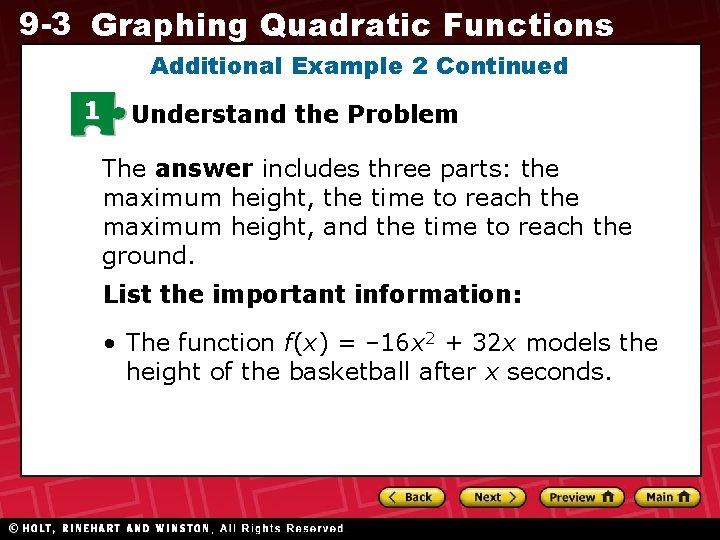9 -3 Graphing Quadratic Functions Additional Example 2 Continued 1 Understand the Problem The answer includes three parts: the maximum height, the time to reach the maximum height, and the time to reach the ground. List the important information: • The function f(x) = – 16 x 2 + 32 x models the height of the basketball after x seconds.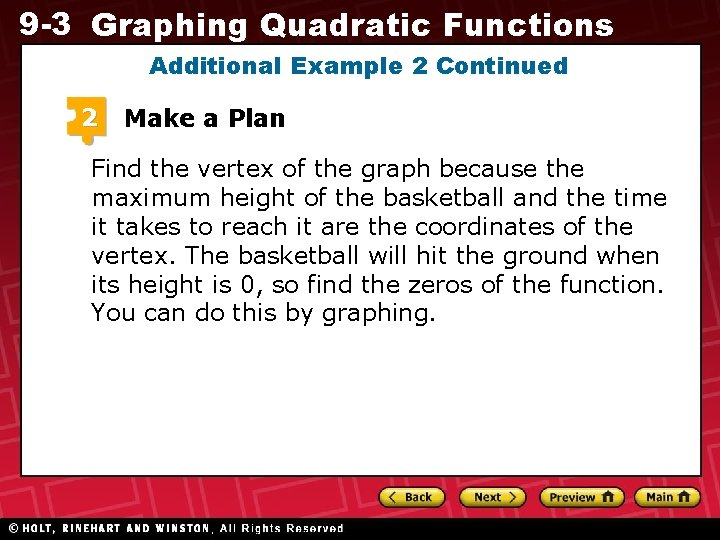9 -3 Graphing Quadratic Functions Additional Example 2 Continued 2 Make a Plan Find the vertex of the graph because the maximum height of the basketball and the time it takes to reach it are the coordinates of the vertex. The basketball will hit the ground when its height is 0, so find the zeros of the function. You can do this by graphing.9 -3 Graphing Quadratic Functions Additional Example 2 Continued 3 Solve Step 1 Find the axis of symmetry. Use x = . Substitute – 16 for a and 32 for b. Simplify. The axis of symmetry is x = 1.9 -3 Graphing Quadratic Functions Additional Example 2 Continued Step 2 Find the vertex. f(x) = – 16 x 2 + 32 x The x-coordinate of the vertex is 1. 2 = – 16(1) + 32(1) Substitute 1 for x. = – 16(1) + 32 = – 16 + 32 Simplify. = The y-coordinate of the vertex is 16. 16 The vertex is (1, 16).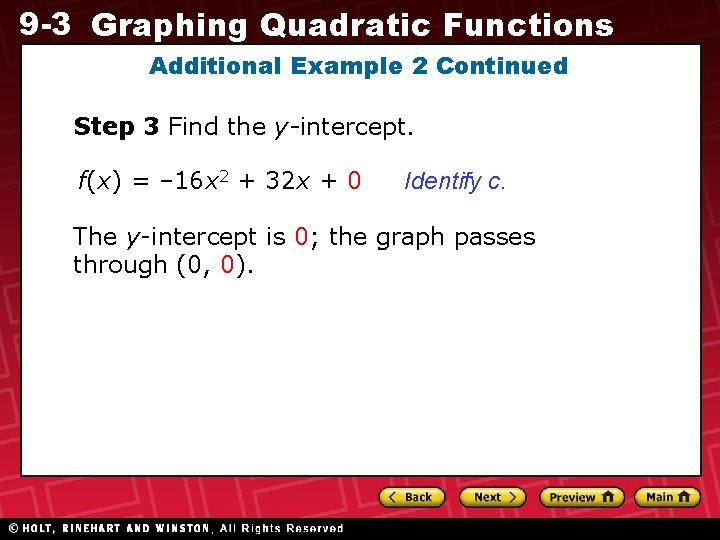9 -3 Graphing Quadratic Functions Additional Example 2 Continued Step 3 Find the y-intercept. f(x) = – 16 x 2 + 32 x + 0 Identify c. The y-intercept is 0; the graph passes through (0, 0).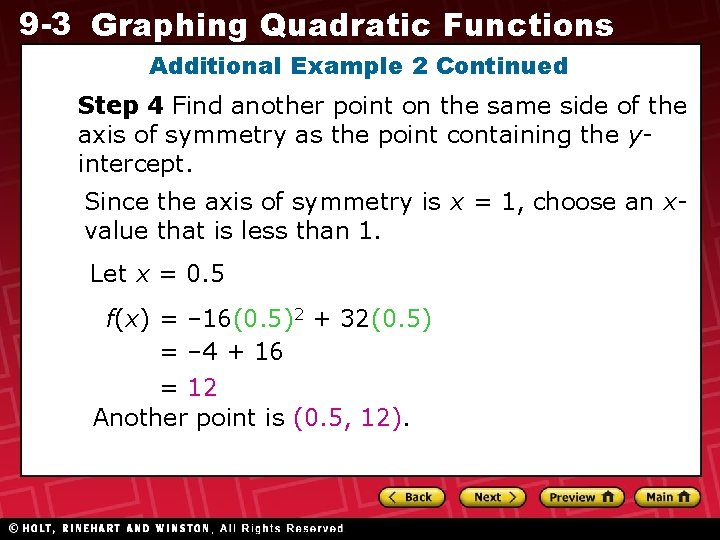9 -3 Graphing Quadratic Functions Additional Example 2 Continued Step 4 Find another point on the same side of the axis of symmetry as the point containing the yintercept. Since the axis of symmetry is x = 1, choose an xvalue that is less than 1. Let x = 0. 5 f(x) = – 16(0. 5)2 + 32(0. 5) = – 4 + 16 = 12 Another point is (0. 5, 12).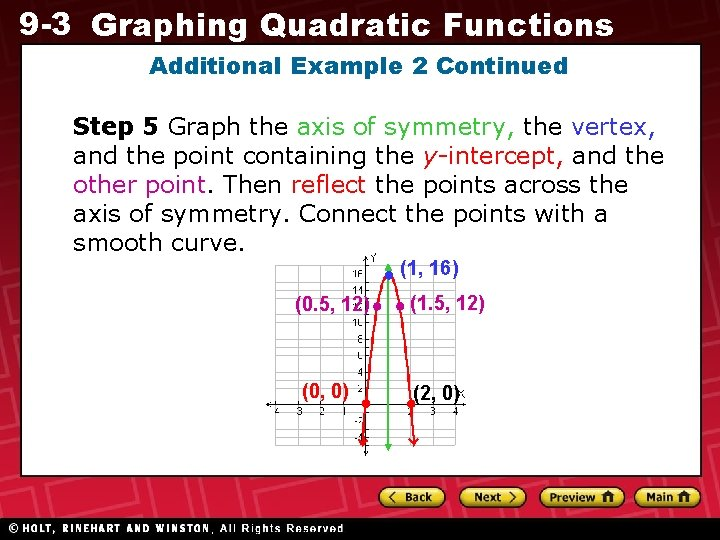9 -3 Graphing Quadratic Functions Additional Example 2 Continued Step 5 Graph the axis of symmetry, the vertex, and the point containing the y-intercept, and the other point. Then reflect the points across the axis of symmetry. Connect the points with a smooth curve. (1, 16) (0. 5, 12) (0, 0) (1. 5, 12) (2, 0)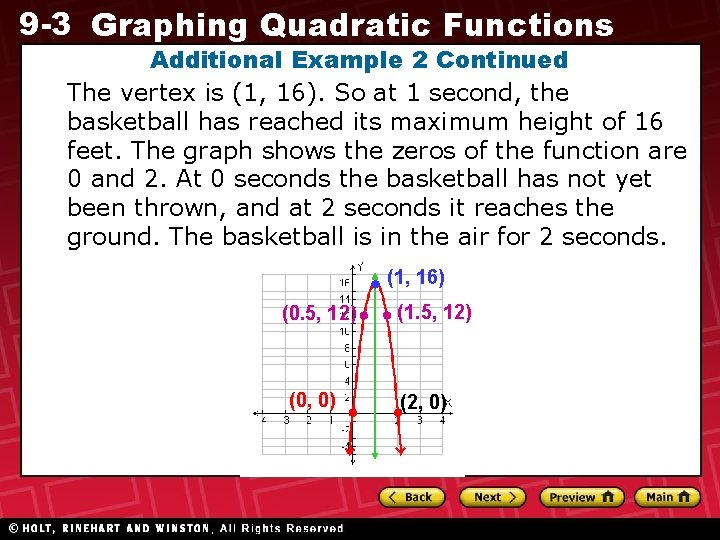9 -3 Graphing Quadratic Functions Additional Example 2 Continued The vertex is (1, 16). So at 1 second, the basketball has reached its maximum height of 16 feet. The graph shows the zeros of the function are 0 and 2. At 0 seconds the basketball has not yet been thrown, and at 2 seconds it reaches the ground. The basketball is in the air for 2 seconds. (1, 16) (0. 5, 12) (0, 0) (1. 5, 12) (2, 0)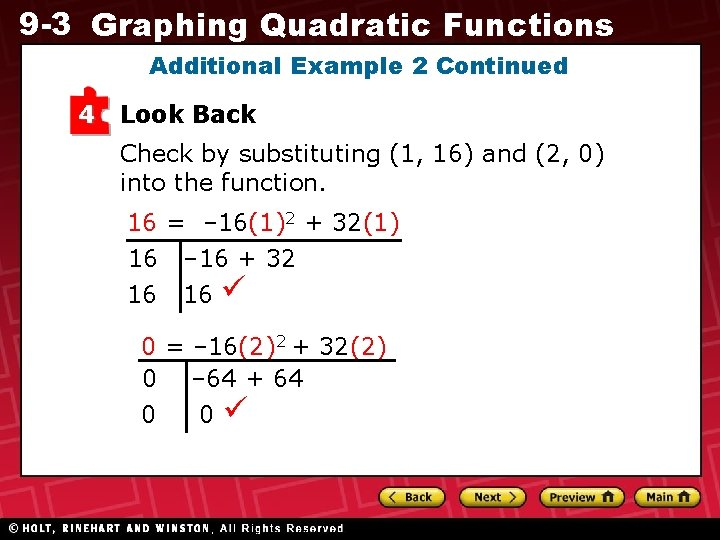9 -3 Graphing Quadratic Functions Additional Example 2 Continued 4 Look Back Check by substituting (1, 16) and (2, 0) into the function. 16 = – 16(1)2 + 32(1) 16 – 16 + 32 16 16 0 = – 16(2)2 + 32(2) 0 – 64 + 64 0 09 -3 Graphing Quadratic Functions Check It Out! Example 2 As Molly dives into her pool, her height in feet above the water can be modeled by the function f(x) = – 16 x 2 + 24 x, where x is the time in seconds after she begins diving. Find the maximum height of her dive and the time it takes Molly to reach this height. Then find how long it takes her to reach the pool.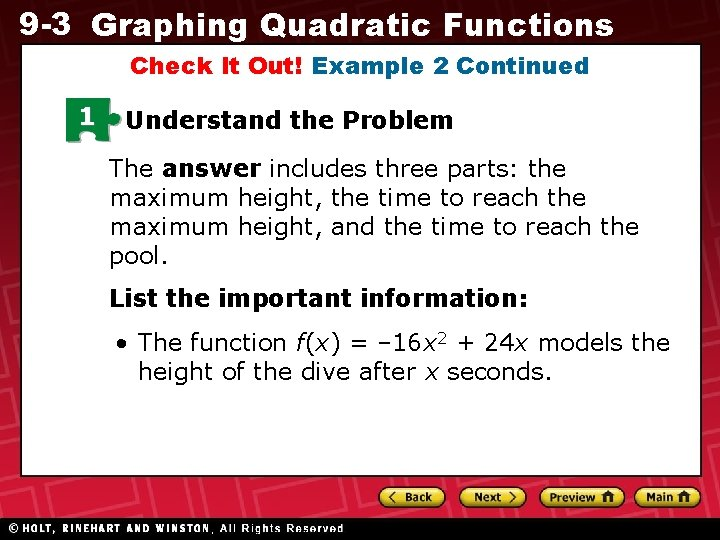9 -3 Graphing Quadratic Functions Check It Out! Example 2 Continued 1 Understand the Problem The answer includes three parts: the maximum height, the time to reach the maximum height, and the time to reach the pool. List the important information: • The function f(x) = – 16 x 2 + 24 x models the height of the dive after x seconds.9 -3 Graphing Quadratic Functions Check It Out! Example 2 Continued 2 Make a Plan Find the vertex of the graph because the maximum height of the dive and the time it takes to reach it are the coordinates of the vertex. The diver will hit the water when its height is 0, so find the zeros of the function. You can do this by graphing.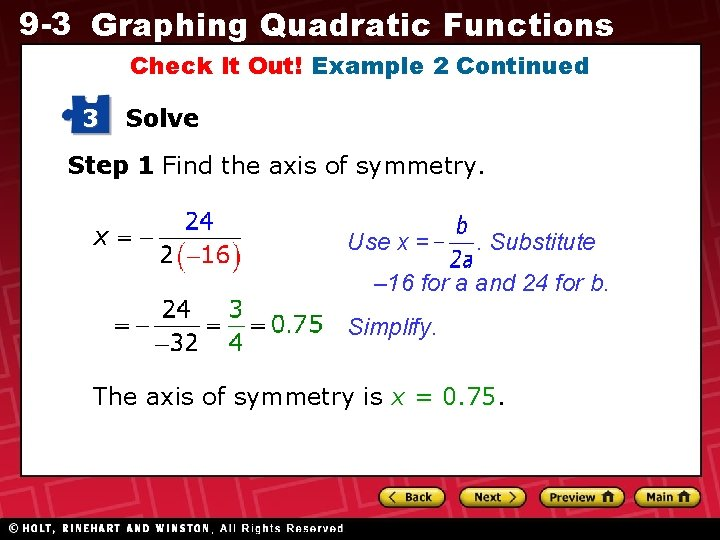9 -3 Graphing Quadratic Functions Check It Out! Example 2 Continued 3 Solve Step 1 Find the axis of symmetry. Use x = . Substitute – 16 for a and 24 for b. Simplify. The axis of symmetry is x = 0. 75.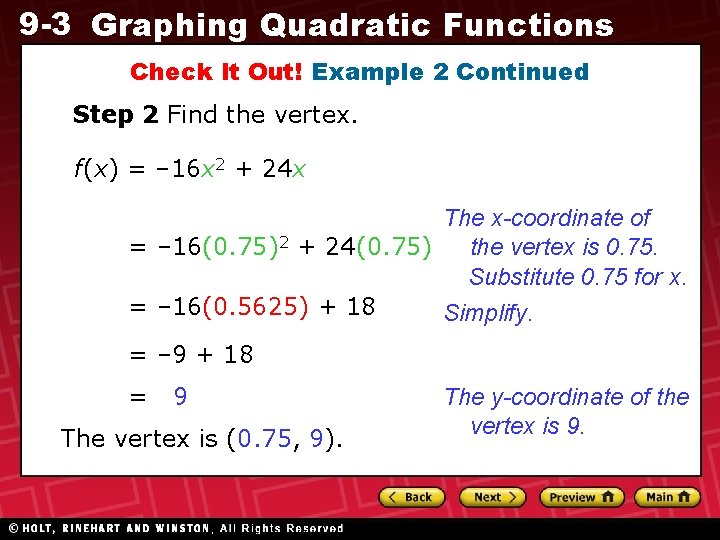9 -3 Graphing Quadratic Functions Check It Out! Example 2 Continued Step 2 Find the vertex. f(x) = – 16 x 2 + 24 x The x-coordinate of the vertex is 0. 75. = – 16(0. 75)2 + 24(0. 75) Substitute 0. 75 for x. = – 16(0. 5625) + 18 Simplify. = – 9 + 18 = 9 The vertex is (0. 75, 9). The y-coordinate of the vertex is 9.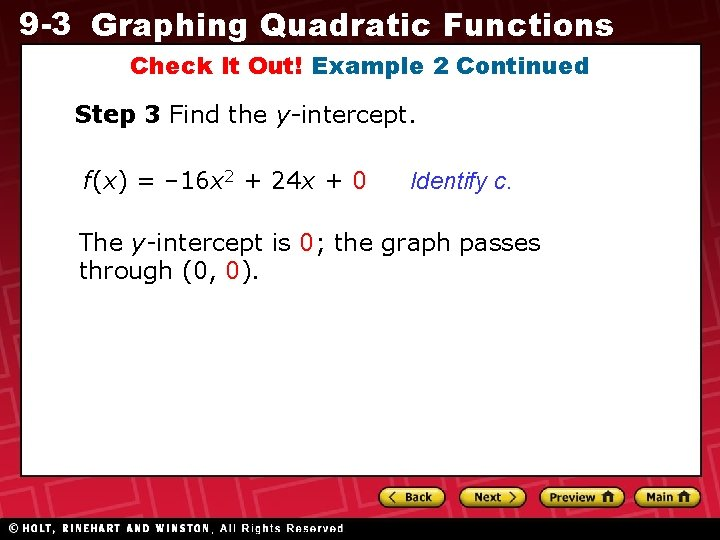9 -3 Graphing Quadratic Functions Check It Out! Example 2 Continued Step 3 Find the y-intercept. f(x) = – 16 x 2 + 24 x + 0 Identify c. The y-intercept is 0; the graph passes through (0, 0).9 -3 Graphing Quadratic Functions Check It Out! Example 2 Continued Step 4 Find another point on the same side of the axis of symmetry as the point containing the y-intercept. Since the axis of symmetry is x = 0. 75, choose an x-value that is less than 0. 75. Let x = 0. 5 f(x) = – 16(0. 5)2 + 24(0. 5) Substitute 0. 5 for x. = – 4 + 12 =8 Another point is (0. 5, 8). Simplify.9 -3 Graphing Quadratic Functions Check It Out! Example 2 Continued Step 5 Graph the axis of symmetry, the vertex, the point containing the y-intercept, and the other point. Then reflect the points across the axis of symmetry. Connect the points with a smooth curve. (0. 75, 9) (0. 5, 8) (0, 0) (1, 8) (1. 5, 0)9 -3 Graphing Quadratic Functions Check It Out! Example 2 Continued The vertex is (0. 75, 9). So at 0. 75 seconds, Molly's dive has reached its maximum height of 9 feet. The graph shows the zeros of the function are 0 and 1. 5. At 0 seconds the dive has not begun, and at 1. 5 seconds she reaches the pool. Molly reaches the pool in 1. 5 seconds. (0. 75, 9) (0. 5, 8) (0, 0) (1, 8) (1. 5, 0)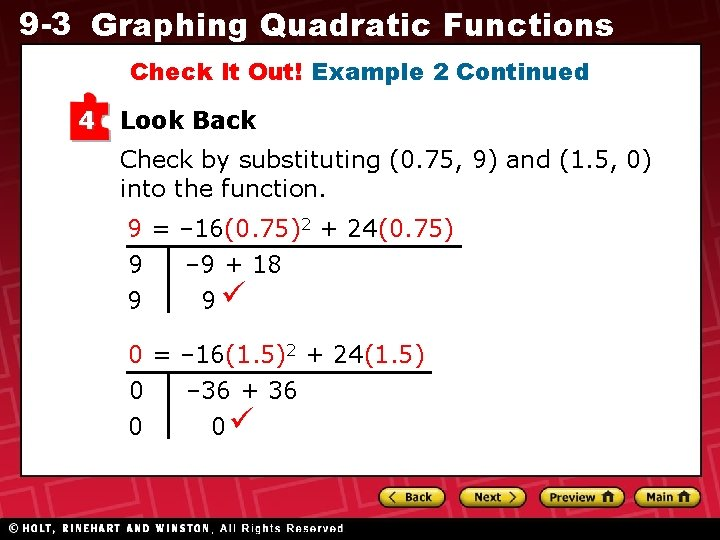9 -3 Graphing Quadratic Functions Check It Out! Example 2 Continued 4 Look Back Check by substituting (0. 75, 9) and (1. 5, 0) into the function. 9 = – 16(0. 75)2 + 24(0. 75) 9 – 9 + 18 9 9 0 = – 16(1. 5)2 + 24(1. 5) 0 – 36 + 36 0 09 -3 Graphing Quadratic Functions Lesson Quiz 1. Graph y = – 2 x 2 – 8 x + 4. 2. The height in feet of a fireworks shell can be modeled by h(t) = – 16 t 2 + 224 t, where t is the time in seconds after it is fired. Find the maximum height of the shell, the time it takes to reach its maximum height, and length of time the shell is in the air. 784 ft; 7 s; 14 s